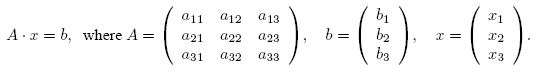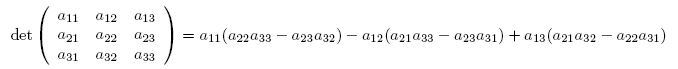Online JudgeProblem SetAuthorsOnline ContestsUser
Web Board
F.A.Qs
Statistical Charts
Problems
Submit Problem
Online Status
Prob.ID:
Register
Authors ranklist
Current Contest
Past Contests
Scheduled Contests
Award Contest
Register
Language:
Cramer's Rule
 Time Limit: 1000MS Memory Limit: 30000K Total Submissions: 3224 Accepted: 778

Description

Background
Consider a system of linear equations, here three equations of three variables x1, x2, x3. The general form looks something like this, with given numbers aij and bi:
a11x1 + a12x2 + a13x3 = b1
a21x1 + a22x2 + a23x3 = b2
a31x1 + a32x2 + a33x3 = b3

Or, using matrices and vectors:According to Cramer's rule, the solution can be given in terms of determinants, i.e.
xi =det(Ai)/det(A)

where Ai is the matrix obtained from A by replacing the i-th column with the vector b. For 3 * 3 determinants,you can use the following simple formula to calculate the determinant:Obviously, Cramer's rule only works for det(A) != 0. One can show that the system has a unique solution if and only if det(A) != 0. Otherwise, the system has either no solution or infinitely many solutions.
Please note that one would not use Cramer's rule to solve a large system of linear equations, simply because calculating a single determinant is as time-consuming as solving the complete system by a more efficient algorithm.
Problem
Given a system of three linear equations in three variables, use Cramer's rule to find the unique solution if it exists. More precisely, calculate the determinants of the Ai and of A and decide by looking at det(A) whether the system has a unique solution. If it does, calculate the solution according to Cramer's rule.

Input

The first line contains the number of scenarios.
For each scenario, you are given three lines corresponding to the three equations, with the coefficients of the matrix A and the coordinates of the vector b arranged as follows:
a11 a12 a13 b1
a21 a22 a23 b2
a31 a32 a33 b3

All numbers are integers in the range {−1000, . . . , 1000}. They are separated by single blanks.

Output

For each scenario print three lines. In the first line, print the determinants of A1, A2, A3, and A, as integers and separated by single blanks. In the second line, print (depending on det(A)) either "No unique solution" or "Unique solution: ", followed by the values of x1, x2, x3 with three digits after the decimal point,again separated from each other by a single blank. For solutions xi with -0.0005 < xi < 0.0005 always print "0.000" instead of the "-0.000" that your print command might come up with. The third line is empty.

Sample Input

3
4 0 0 1
0 2 0 2
0 0 1 4
1 2 3 1
1 1 1 2
2 2 2 3
1 0 0 1
0 1 0 0
0 0 -1 0

Sample Output

2 8 32 8
Unique solution: 0.250 1.000 4.000

1 -2 1 0
No unique solution

-1 0 0 -1
Unique solution: 1.000 0.000 0.000

Source

TUD Programming Contest 2001, Darmstadt, Germany

[Submit]   [Go Back]   [Status]   [Discuss]Home PageGo BackTo top